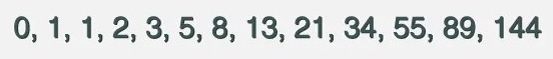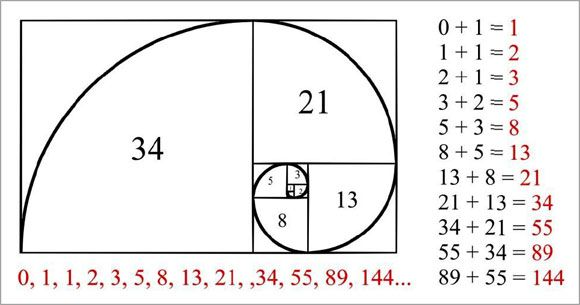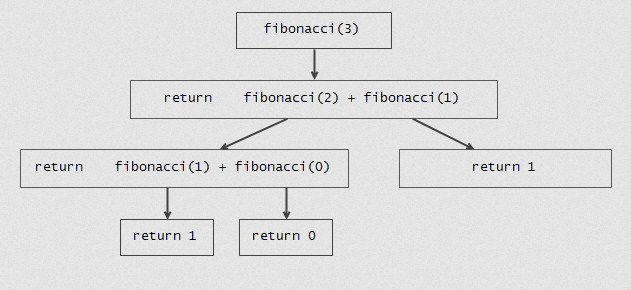# C Program to Generate Fibonacci Series

In this tutorial you will learn about the C Program to Generate Fibonacci Series and its application with practical example.

In this tutorial, we will learn to generate Fibonacci series using C programming language. We will show you two different method to generate Fibonacci Series in C programming:
1) Fibonacci Series without recursion
2) Fibonacci Series using recursion

## Prerequisites

Before starting with this tutorial we assume that you are best aware of the following C programming topics:

## What Is Fibonacci Series?

The Fibonacci series is a sequence of numbers where the next numbers is the sum of the previous two numbers in the series. The first two numbers of the Fibonacci sequence are 0 followed by 1.

Example:-In the example above, 0 and 1 are the first two terms of the Fibonacci series. The third term of series is evaluated by adding the previous two terms. In this case 0 and 1 are the previous two terms so by adding them (0+1 = 1) we get 1 as the third term of series. Similarly, the next term is evaluated by adding second and third term of the series. This process is repeated until the number of the terms requested is reached. Please have look at the image below to understand the process.## Print Fibonacci Series Without Recursion

In this program we will print fibonacci series using for loop. We would first declared and initialized the required variables. Next, we would prompt user to input the number of terms. Later we will generate the fibonacci series of specified number of terms.

Output:-

In the above program, we have first declared and initialized a set variables required in the program.

• n = number of terms to be printed
• ctr = counter for iteration
• t1= term 1, initialized with 0
• t2= term 2, initialized with 1
• t3 = next term to be computed

In the next statement user will be prompted to enter the number of terms which will be assigned to variable ‘n’. Now we will loop through the iterator ‘ctr’ upto the number of terms. Inside the for loop we are first printing the value of t1(initialized with 0) then in the next statement we are computing the next term(t3) value by adding previous two terms that is t1 and t2. Then we are assigning new values to t1 and t2 as to hold previous two terms value for another next term. This will be repeated until it print the fibonacci series of required number of terms.

## Print Fibonacci Series Using Recursion

In this program we will print fibonacci series using a recursive function. A function is called recursive when calls itself. It very important to have termination condition in recursive function. Please take look at the example image below, to understand the process of evaluating fibonacci series of 3 terms using a recursive function.Example:-

Output:-

In the above program, we have first declared a function called as fibonacci that will recursively generate the fibonacci terms. Later in the main function, we declared required variables.

• n = number of terms to be printed
• t = hold the term value, initialized with 0
• i = for iteration

In the next statement user will be prompted to enter the number of terms which will be assigned to variable ‘n’. Now we will loop through the iterator ‘i’ upto the number of terms. Inside the loop we are calling fibonacci function to return fibonacci terms recursively upto the number of terms.

Next, we have defined the fibonacci function. Inside the function, we first check if the number n is zero or one. If yes, we return the value of n. If not, we recursively call fibonacci function with the values n-1 and n-2 until it reach to a terminating condition.

In this tutorial we have learn about the C Program to Generate Fibonacci Series and its application with practical example. I hope you will like this tutorial.